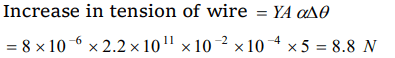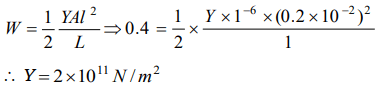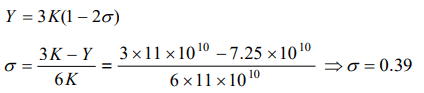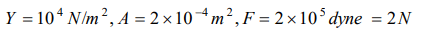## Mechanical Properties of Solids Questions and Answers Part-5

1. After effects of elasticity are maximum for
a) Glass
b) Quartz
c) Rubber
d) Metal

Explanation: Glass

2. In suspended type moving coil galvanometer, quartz suspension is used because
a) It is good conductor of electricity
b) Elastic after effects are negligible
c) Young's modulus is greater
d) There is no elastic limit

Explanation: Elastic after effects are negligible

3.A force of 200 N is applied at one end of a wire of length 2 m and having area of cross-section $10^{-2}cm ^{2}$. The other end of the wire is rigidly fixed. If coefficient of linear expansion of the wire $\alpha=8\times10^{-6}\diagup^{\circ}C$    and Young's modulus $Y=2.2\times10^{11} N\diagup m^{2}$    and its temperature is increased by 5°C, then the increase in the tension of the wire will be
a) 4.2 N
b) 4.4 N
c) 2.4 N
d) 8.8 N

Explanation:4. When compared with solids and liquids, the gases have
a) Minimum volume elasticity
b) Maximum volume elasticity
c) Maximum Young's modulus
d) Maximum modulus of rigidity

Explanation: A small change in pressure produces a large change in volume.

5. The length of a wire is 1.0 m and the area of cross-section is $1.0\times10^{-2} c m^{2}$ . If the work done for increase in length by 0.2 cm is 0.4 joule, then Young's modulus of the material of the wire is
a) $2.0\times10^{10} N\diagup m^{2}$
b) $4\times10^{10} N\diagup m^{2}$
c) $2.0\times10^{11} N\diagup m^{2}$
d) $2\times10^{10} N\diagup m^{2}$

Explanation:6. The quality of the material which opposes the change in shape, volume or length is called
a) Intermolecular repulsion
b) Intermolecular behaviour
c) Viscosity
d) Elasticity

Explanation: Elasticity

7. For silver, Young's modulus is $7.25\times10^{10} N\diagup m^{2}$    and Bulk modulus is $11\times10^{10} N\diagup m^{2}$  . Its Poisson's ratio will be
a) – 1
b) 0.5
c) 0.39
d) 0.25

Explanation:8. The longitudinal strain is only possible in
a) Gases
b) Fluids
c) Solids
d) Liquids

Explanation: Solids

9.If the density of the material increases, the value of Young's modulus
a) Increases
b) Decreases
c) First increases then decreases
d) First decreases then increases

10. Young's modulus of rubber is $10^{4} N\diagup m^{2}$  and area of cross-section is $2cm^{2}$ . If force of $2\times10^{5} dynes$    is applied along its length, then its initial length l becomes